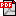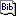SPONSORSCOLLABORATIONS### CLaMPI - a Caching LAyer for MPI

#### Motivation

The constantly increasing gap between communication and computation performance emphasizes the importance of communication-avoidance techniques. Caching is a well-known concept used to reduce accesses to slow local memories. In this work, we extend the caching idea to MPI-3 Remote Memory Access (RMA) operations. Here, caching can avoid inter-node communications and achieve similar benefits for irregular applications as communication-avoiding algorithms for structured applications. We propose CLaMPI, a caching library layered on top of MPI-3 RMA, to automatically optimize code with minimum user intervention. We demonstrate how cached RMA improves the performance of a Barnes Hut simulation and a Local Clustering Coefficient computation up to a factor of 1.8x and 5x, respectively. Due to the low overheads in the cache miss case and the potential benefits, we expect that our ideas around transparent RMA caching will soon be an integral part of many MPI libraries.

#### Caching-Enabled Windows

CLaMPI offers three different strategies (i.e., operational modes) to enable the caching of RMA accesses: transparent, always-cache, and user-defined.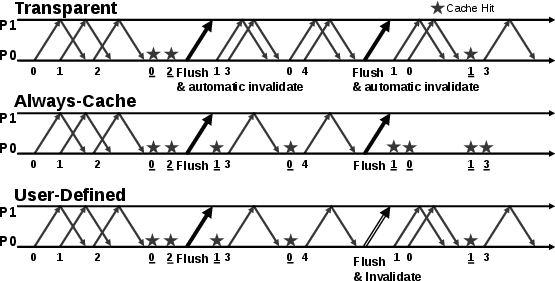Transparent It allows applications to enable caching without any code change. All the MPI windows are caching-enabled. Since no assumptions can be made on the data access pattern, the cache is invalidated at each epoch closure.

Always-Cache If the memory area identified by the window is read-only for the entire window lifespan then there is no need to perform any cache invalidation. Examples of such application are the ones applying graph-processing algorithm: if the graph structure is not modified, then the window representing it can be set in the always-cache mode. Such information can be communicated to CLaMPI as an MPI_Info key passed at window creation time. - i.e., as argument to MPI_Win_create or MPI_Win_allocate.

User-Defined This strategy let the user define epochs, or sets of consecutive epochs, in which the memory area identified by a window is in a read-only state. Use cases that can take advantage of this operational mode are, for example, BSP-like (Bulk Synchronous Parallel) applications presenting steps where no write accesses are performed towards the specific window. The Barnes-Hut algorithm falls in this category. With this strategy, the user creates the window with the always-cache option. The cache can be explicitly invalidated using the CMPI_Win_invalidate(MPI_Win win) call when the sequence of read-only epochs terminates.

#### Adaptive scheme: dynamically adjust the cache parameters

To cache entries CLaMPI uses a hash table as index (with Cuckoo hashing) and a memory buffer as storage. The sizes of the index and the memory buffer play an important role for the caching efficiency (i.e., number of hits, evictions, etc.). The optimal value of these parameters can be heavily dependent on the specific application and the settings with which this application is ran, and also impacts its performance.

CLaMPI allows to set the default value of these parameters at compile time. Such values can be overridden at running time by using specific environment variables. So they can be adjusted for each application/settings.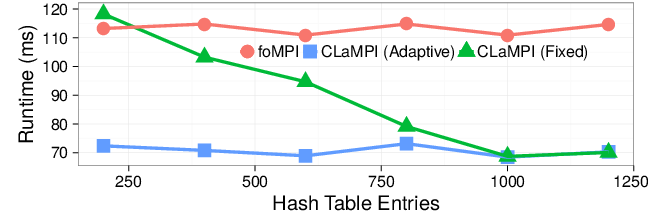However, there are two problems: Who knows the optimal values of these parameter for the application that we want to run? Also, we need to consider that the behavior of the application can change while running: by fixing these parameters we could over- or under-estimate the actual needs of the application. What if the application's (cache) behavior changes at running time?

CLaMPI implements an adaptive scheme that adjusts the index and memory buffer sizes dynamically, taking track of a set of performance/efficiency indicators. Here we show the results of a benchmark comparing the same access pattern with and without adaptive scheme. Details of this benchmark can be found in the paper (Section IV.A). The fixed strategy presents poor performance when the index size is small w.r.t. the number of distinct gets due to high number of conflicts. This does not happen with the adaptive strategy, which is able to adjust the hash table size at runtime, minimizing the number of conflicts.

#### Barnes-Hut with CLaMPI

The N-Body problem consists of simulating the evolution of a system composed by N bodies. The system evolves as the bodies apply their own force on the others. The algorithm operates on discrete time intervals: at each time step the forces applied from one body on all the others are computed, updating the position and velocity of the bodies. A naive approach to this problem would lead to an $O(N^2)$ algorithm. The Barnes-Hut algorithm presents $O(N \cdot logN)$ time complexity.

We modified the UPC implementation by Larkins et al. introducing MPI One Sided operations for retrieving/storing cells and leaves of the tree. We enable CLaMPI with the user-defined mode, that allows us to invalidate the cache after the termination of the force computation phase.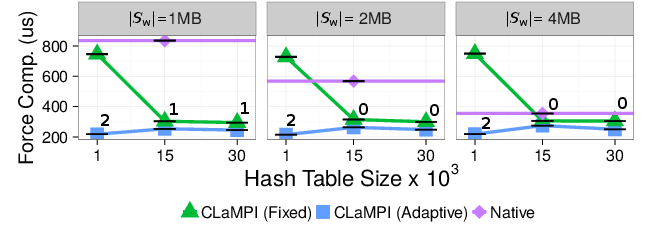We compare the two CLaMPI strategies, adaptive and fixed, with an ad-hoc caching system, referred to as native, that was included in our reference UPC implementation. The adaptive strategy is annotated with the number of performed invalidations/adjustments. The Force Computation time required by foMPI is $1.53$ms. The experiment fixes the number of processing elements to $P=16$ and the total number of bodies to $n=20$K. The CLaMPI parameters - such as index and memory buffer size - are varied in order to find the best setting. The memory size of the native caching solution is set to the same value of the CLaMPI's one. The adaptive strategy is the one presenting the best performance: in all the cases it converges to $1MB$ as memory buffer size, while it sets the length of the index to $20$K entries (averaged on all the processes). With an index of $1K$ entries, the performance of the fixed strategy is limited by the high number of conflicts, The native solution performance heavily depends on its memory size: it ranges from $820us$ with $1$MB to $400us$ with $4$MB memory buffer size. This behavior can be explained by the fact that this system is a block-based software cache with direct mapping, hence the number of conflicts is strictly related to the available memory size.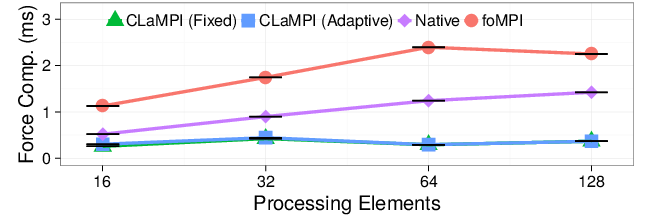At the right we show the results of a weak scaling experiment. The index and the memory buffer sizes are set to 30K entries and 2MB, respectively.These are the initial parameters of the adaptive strategy. The number of bodies is set to $N = P \cdot 8K$ and $P$, that is the number of processing elements, varies between $16$ and $128$. Both the CLaMPI strategies outperform the native and foMPI solutions up to a factor of $\sim3$x and $\sim5$x, respectively. With this settings, the adaptive strategy does not perform any adjustment of the initial parameters.

#### Local Clustering Coefficient (LCC) with CLaMPI

The local clustering coefficient of a vertex (node) in a graph quantifies how close its neighbors are to being a clique (complete graph). Duncan J. Watts and Steven Strogatz introduced the measure in 1998 to determine whether a graph is a small-world network (quoted from Wikipedia).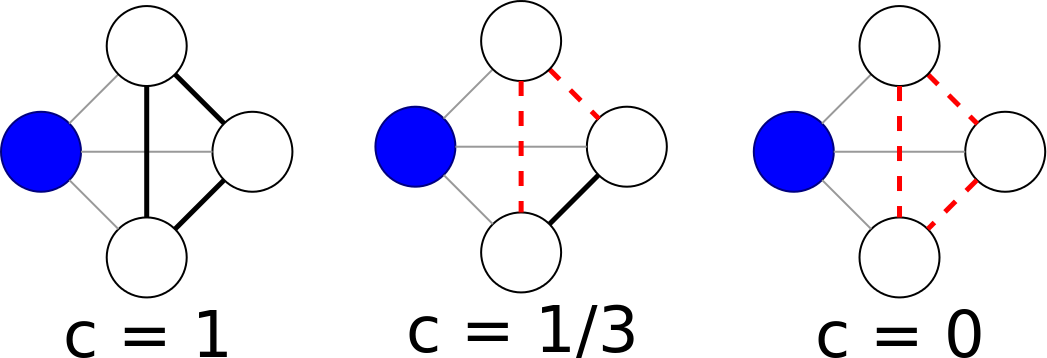We introduce CLaMPI in an LCC algorithm leveraging one-sided operations. Given an input graph, to compute the LCC of a local vertex $v$, a process $p_i$ has to retrieve the adjacency list of every incident vertex $u$. If the owner of $u$ is not $p_i$, the retrieve operation can be performed as an RMA get. The number of gets issued by a single process depends on the size of its own partition and on the degree of the vertices in such partition. The size of the each issued get depends on the degree of each neighbor of the vertex $v$. The LCC computation exposes data reuse since the adjacency list of the same vertex $u$ can be accessed several times by a single process: every time $u$ appears in the adjacency list of an owned node.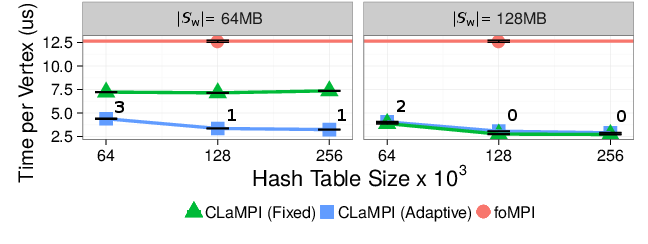On the right, we compare the vertex processing time of the CLaMPI adaptive and fixed configurations with respect to foMPI. The adaptive strategy is annotated with the number of performed invalidations/adjustments. The CLaMPI fixed strategy with a memory buffer of $64$MB is limited by the significant number of evictions and failed accesses. Increasing the memory buffer size the number of evictions and failed accesses decreases, explaining the $5$x speedup presented by the fixed strategy with respect to foMPI when the memory buffer size is set to $128M$MB and the index size to $256$K entries. The adaptive strategy achieves a speedup similar to the one presented by the best fixed configuration, independently from the starting parameters.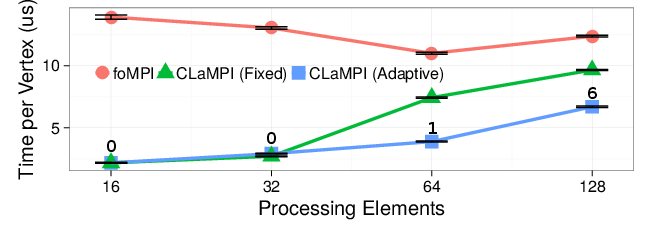On the left we report the results of the weak scaling experiment, where the problem size per processing element stays constant. A detailed description of the benchmark can be found in the paper. The adaptive strategy is annotated with the total number of automatically performed invalidations/adjustments. Increasing the graph scale with the number of processes leads to a situation in which the number of get per process stays constant but the average get size increases. As a consequence, the fixed strategy suffers of an higher number of evictions and failed accesses when increasing the number of processing elements. This explains the gap between the fixed and the adaptive strategy when $P>32$. In fact, the adaptive strategy is able to resize the memory buffer size in order to accommodate the larger gets,

#### Library Interface

To facilitate benchmarking and comparison with standard MPI implementations, we decide to prefix the CLaMPI function with CMPI. Please note that, except for that, they keep the same signature of the correspondent MPI functions.

Window Creation
The CLaMPI window mode can be set as follows:

CMPI_Win cmpi_win;
MPI_Info info;
MPI_Info_create(&info);
MPI_Info_set(info, CLAMPI_MODE, CLAMPI_USER_DEFINED);

CLAMPI_MODE can be set to: CLAMPI_TRANSPARENT (default), CLAMPI_ALWAYS_CACHE, and CLAMPI_USER_DEFINED.

The window can be created with
int CMPI_Win_create(void * base, MPI_Aint size, int disp_unit, MPI_Info info, MPI_Comm comm, CMPI_Win * win);

or
int CMPI_Win_allocate(MPI_Aint size, int disp_unit, MPI_Info info, MPI_Comm comm, void * baseptr, CMPI_Win * win);


Performing Get Operations
CMPI_Get performs the get by forwarding it to CLaMPI.
int CMPI_Get(void * origin_addr, int origin_count, MPI_Datatype origin_datatype, int target_rank, MPI_Aint target_disp, int target_count, MPI_Datatype target_datatype, CMPI_Win win);


Window Flushing and Invalidating
Flushing a window will lead to its automatic invalidation when using the CLAMPI_TRANSPARENT window mode.
int CMPI_Win_flush(int rank, CMPI_Win win);

Instead, the window needs explicit invalidation when the CLAMPI_USER_DEFINED mode is chosen.
int CMPI_Win_invalidate(CMPI_Win win);


Cleaning Up
The window can be freed with:
int CMPI_Win_free(CMPI_Win * win);


#### Download

The latest version can be found in the CLaMPI GitHub repository
Additionally, you can download the latest stable version here:

 Version Date Changes libclampi-1.0.tar.gz - (367 KB) April 30, 2017 First release

#### References

 IPDPS'17  S. Di Girolamo, F. Vella and T. Hoefler: Transparent Caching for RMA Systems In Proceedings of the 31st IEEE International Parallel & Distributed Processing Symposium (IPDPS'17), presented in Orlando, FL, USA, IEEE, May 2017, (acceptance rate: 22%, 116/516)